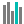#MEDIAN (PQL - xl)

This function returns the median, the number in the middle of the provided set of numbers; that is, half the numbers have values that are greater than the median, and half have values that are less than the median

• Library: PQL \ Spreadsheet \ Statistical
• Compatibility: Any content (regardless of data source) in the Tabulate spreadsheet module
• Solve: This function can be used with the Solve plug-in: both as part of the objective function and constraint functions

#### Syntax

MEDIAN(number1, number2)

##### Function Arguments
 Name Description Type Optional number1 Double-precision floating-point value, an integer value, or an array (cell range) of these StringOrNumber number2 Double-precision floating-point value, an integer value, or an array (cell range) of these StringOrNumber